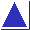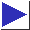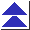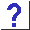# comp.lang.c FAQ list ·Question 14.4a

Q: My floating-point calculations are acting strangely and giving me different answers on different machines.

A: First, see question 14.2.

If the problem isn't that simple, recall that digital computers usually use floating-point formats which provide a close but by no means exact simulation of real number arithmetic. Among other things, the associative and distributive laws do not hold completely; that is, order of operation may be important, and repeated addition is not necessarily equivalent to multiplication. Underflow, cumulative precision loss, and other anomalies are often troublesome.

Don't assume that floating-point results will be exact, and especially don't assume that floating-point values can be compared for equality. (Don't throw haphazard ``fuzz factors'' in, either; see question 14.5.) Beware that some machines have more precision available in floating-point computation registers than in double values stored in memory, which can lead to floating-point inequalities when it would seem that two values just have to be equal.

These problems are no worse for C than they are for any other computer language. Certain aspects of floating-point are usually defined as ``however the processor does them'' (see also questions 11.33 and 11.34), otherwise a compiler for a machine without the ``right'' model would have to do prohibitively expensive emulations.

This document cannot begin to list the pitfalls associated with, and workarounds appropriate for, floating-point work. A good numerical programming text should cover the basics; see also the references below. (Beware, though, that subtle problems can occupy numerical analysts for years.)

References: Kernighan and Plauger, The Elements of Programming Style Sec. 6 pp. 115-8
Knuth, Volume 2 chapter 4
David Goldberg, ``What Every Computer Scientist Should Know about Floating-Point Arithmetic''